## General Question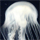# A ball thrown straight up in the air at 70 m/s, how high does the ball go?

Asked by jdogg (871) February 8th, 2010

I need some help with physics, do you know the answer and how to solve it? Thanks. :)

Observing members: 0Composing members: 0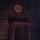you need to factor in gravity which is -9.82 m/s i dont remember how to do it though

JONESGH (3554)“Great Answer” (0) Flag as…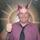i would say the question can not be answered. im no physicist, but surely you need to know how long it is thrown up for.

poisonedantidote (21643)“Great Answer” (1) Flag as…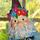I am assuming we are neglecting air resistance.

V final = V initial + at, where a is acceleration due to gravity and t is time.
Acceleration on Earth is -9.81 m/s2. V initial is 70 m/s. Logic tells us that the ball will reach a certain maximum height then fall back to the ground because of gravity. At this maximum (which is when the ball will have traveled the furthest), you should know that the velocity is zero for an instant. Therefore, we know V final, that is the velocity at the maximum point is zero. Plugging all knowns into the equation, you can solve for time it takes the ball to reach this distance which is 7.14 seconds.

X final = ½ at2 + vt + x initial, where X final is the distance you are seeking, a, t, and v are as before, and x initial is the initial position of the ball. Let us define the position of the ball such that its initial position is zero to keep things simple. Therefore, the equation reduces to:

X final = ½ at2 + vt = ½ (-9.81) (7.14)^2 + (70) (7.14) = 249.7 m

Alternatively, you can use the average velocity to calculate the distance traveled. This is (70 + 0) / 2, or 35 m/s. Multiply this by the time we calculated above and you get the same distance as above.

If this is a homework problem, you had better start paying attention in class or you’ll be screwed. This is the basic, easy, stupid stuff that you’re supposed to learn in high school; it gets much more complicated. You also need to know how to derive the equations used above to really understand what is going on.

lilikoi (10079)“Great Answer” (5) Flag as…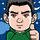The time to the top of throw before returning to earth:

Time = (Velocity final – Velocity initial) / Acceleration

Because we are finding how long it took the ball to reach it’s highest point, the final velocity should be 0. Initial velocity is given, and acceleration due to gravity is 9.8 m/s

Time = (0 m/s – 70 m/s ) / -9.8 m/s
Time = -70 m/s / -9.8 m/s
Time = 7.14 s

Distance traveled:

Distance = Speed * Time
Distance = (70 m/s)*(7.14 second)
Distance = 499.8 meters

I’m not sure how I got a different answer than @lilikoi

MebiByte (660)“Great Answer” (0) Flag as…@MebiByte You didn’t take into consideration the action of gravity on the ball, as the ball is thrown straight up.

lilikoi (10079)“Great Answer” (0) Flag as…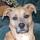All the way up to its apogee. And not one inch farther.

CyanoticWasp (20070)“Great Answer” (0) Flag as…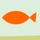None of you seem to be including the action of friction.

How big is the ball? How much does it weigh? Does it have dimples?

grumpyfish (6630)“Great Answer” (2) Flag as…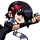Roughly 249 meters.

Ivan (13439)“Great Answer” (0) Flag as…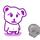what is the mass

dr34m3r (308)“Great Answer” (0) Flag as…@dr34m3r I’d assume since they said “ball” and not “small asteroid” that the mass of the ball is sufficiently small. =)

grumpyfish (6630)“Great Answer” (0) Flag as…@grumpyfish XD. kinetics… i didnt do so well on that unit…. plus i didnt really think it.

first thing that came to mind-

v=dt LOLOL XD

dr34m3r (308)“Great Answer” (0) Flag as…I believe this is actually kinematics…which differs from kinetics…

lilikoi (10079)“Great Answer” (0) Flag as…or